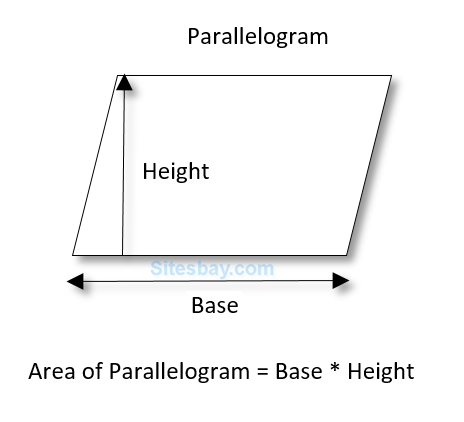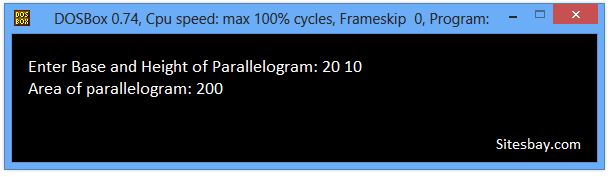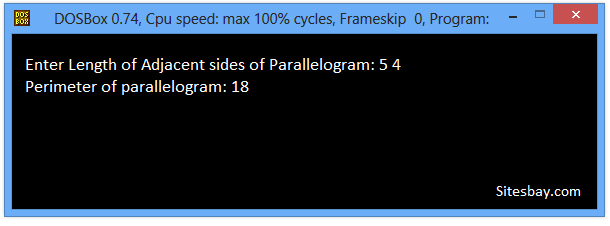# Find Area of Parallelogram Program in C++

## C++ Program to Find Area of Parallelogram

A parallelogram is quadrilaterals(having four sides) with opposite sides parallel and equal in length. Opposite angles of a parallelogram are also equal.

To calculate the area of parallelogram we need length of Base and Height. We can choose any side of a parallelogram as base to calculate area of parallelogram. Height of a parallelogram is the perpendicular distance between the base and it's opposite side.## Formula

```Area of Parallelogram = B X H

Where,
B is the length of base of parallelogram.
H is the length of height of parallelogram.
```

## C++ Program to Find Area of Parallelogram

```#include<iostream.h>
#include<conio.h>

void main()
{
float base, height, area;
clrscr();
cout<<"Enter Base and Height of Parallelogram: ";
cin>>base>>height;

// Area of parallelogram = base X height
area = base * height;
cout<<"Area of parallelogram: "<<area;
getch();
}
```

## Output

```Enter Base and Height of Parallelogram: 20 10
Area of parallelogram: 200
```## C++ Program to Find Perimeter of Parallelogram

The perimeter of a parallelogram can be calculated by adding the length of all four sides of parallelogram. As we know the length of opposite sides of parallelogram are equal, we can add any two adjacent sides of parallelogram and then multiply it with 2 to get perimeter of parallelogram.

## Formula

```Perimeter of parallelogram
Perimeter of Parallelogram = 2X(S1 + S2)
Where, S1 and S2 are length of adjacent sides of parallelogram.
```

## C++ Program to Find Perimeter of Parallelogram

```#include<iostream.h>
#include<conio.h>

void main()
{
float side1, side2, perimeter;
clrscr();
cout<<"Enter Length of Adjacent sides of Parallelogram: ";
cin>>side1>>side2;
// Perimeter of parallelogram = 2X(side1 + side2)
perimeter = 2*(side1 + side2);
cout<<"Perimeter of parallelogram: "<<perimeter;

getch();
}
```

## Output

```Enter Length of Adjacent sides of Parallelogram: 5 4
Perimeter of parallelogram: 18
```## Pure VPN Privide Lowest Price VPN Just @ \$1.65. Per Month with Non Detected IP Lowest Price Non Detected IP VPN